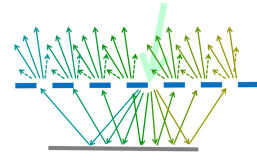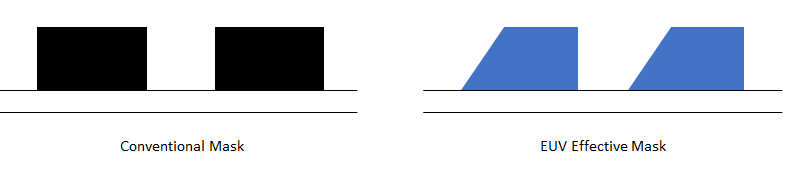```WP_Term Object
(
[term_id] => 16126
[name] => Lithography
[slug] => lithography
[term_group] => 0
[term_taxonomy_id] => 16126
[taxonomy] => category
[description] =>
[parent] => 0
[count] => 129
[filter] => raw
[cat_ID] => 16126
[category_count] => 129
[category_description] =>
[cat_name] => Lithography
[category_nicename] => lithography
[category_parent] => 0
)
```

# Double Diffraction in EUV Masks: Seeing Through The Illusion of Symmetry

Double Diffraction in EUV Masks: Seeing Through The Illusion of Symmetry
by Fred Chen on 05-22-2022 at 7:00 am

At this year’s SPIE Advanced Lithography conference, changes to EUV masks were particularly highlighted, as a better understanding of their behavior is becoming clear. It’s now confirmed that a seemingly symmetric EUV mask absorber pattern does not produce a symmetric image at the wafer, as a conventional DUV mask would [1, 2]. The underlying reason for this is the mask is illuminated by a spread of angles skewed to one side of the vertical axis. Each angle has a different reflection from the multilayer of the EUV mask. Moreover, the EUV light is diffracted twice, one time before entering the multilayer, and a second time after exiting the multilayer (Figure 1).Figure 1. Double diffraction in an EUV mask. Each color represents a particular family of diffraction orders originating from one reflected diffraction order from the first diffraction of light entering the multilayer.

The first diffraction from an array of lines on the EUV mask with pitch p produces a Fourier series of the form

… + A_1 exp[-i 2pi x/p] + A0 + A1 exp[i 2pi x/p] +…

Each series term An exp [i 2pi n x/p] represents a diffraction order propagating in a given direction. Each propagating direction leads to a different multilayer reflectance Rn for that order.

… + A_1 R_1 exp[-i 2pi x/p] + A0 R0 + A1 R1 exp[i 2pi x/p] +…

Passing through the array again when exiting the multilayer, each order generates a second set of diffraction orders, with their amplitudes labeled by B instead of A. The directions of these second sets of diffraction order overlap those of the first. By combining the waves coherently propagating in the same directions, we get:

… + [… + A_1 R_1 B0 + A0 R0 B_1 + A1 R1 B_2 + …] exp[-i 2pi x/p] + [… + A_1 R_1 B1 + A0 R0 B0 + A1 R1 B_1 + …] + [… + A_1 R_1 B2 + A0 R0 B1 + A1 R1 B0 + …] exp[i 2pi x/p] + …

Since the mask structures in the array are symmetric as fabricated, we may approximate A1 = A_1, B1 = B_1. However, R1 does not qual R_1, as they correspond to different angles, and even a seemingly small angular difference can drop the reflectance significance. With this consideration, when we compare the -1st harmonic amplitude [… + A_1 R_1 B0 + A0 R0 B_1 + A1 R1 B_2 + …] with the 1st harmonic amplitude [… + A_1 R_1 B2 + A0 R0 B1 + A1 R1 B0 + …], they are not equal. In a symmetric structure that a rectangular profile is expected to be, these should be equal. However, physically, the structure is not behaving so much as a rectangular wave profile but more like a trapezoidal wave profile, largely due to the shadowing effect from the off-axis incident direction of the light (Figure 2). The effective slope of the sidewall is dependent on the shadowing, and hence, the illumination angle.Figure 2. (Left) A conventional photomask provides a symmetric absorption profile. (Right) On the other hand, for an EUV mask, the trapezoidal profile is a more accurate depiction of the asymmetry effects from shadowing, even though the EUV mask structures themselves are symmetric as fabricated. The illumination angle(s) will determine the shadowing, and hence, the effective slope angle.

Relying on a trapezoidal absorption profile for the mask is a notable departure from the symmetric rectangular profile that has always been used in conventional DUV photomasks. It causes the numerous anomalies of EUV imaging that have already been published over the many years of EUV development. As EUV lithography especially makes more use of dipole illumination (beams coming from two different angles), the angle asymmetry between +1st and -1st diffraction orders becomes more relevant .

#### References

 C. van Lare, F. Timmermans, J. Finders, “Mask-absorber optimization: the next phase,” J. Micro/Nanolith. MEMS MOEMS 19, 024401 (2020).

 A. Erdmann, H. Mesilhy, P. Evanschitzky, “Attenuated phase shift masks: a wild card resolution enhancement for extreme ultraviolet lithography?,” J. Micro/Nanolith. MEMS MOEMS 21, 020901 (2022).

Demonstration of Dose-Driven Photoelectron Spread in EUV Resists

Adding Random Secondary Electron Generation to Photon Shot Noise: Compounding EUV Stochastic Edge Roughness

Intel and the EUV Shortage